## Detecting Barometer sensor in PSLab Android App

The Pocket Science Lab Android app has Barometer Instrument implemented in it. Although the instrument  is currently working through the mobile sensors and not the PSLab i2c library as there were some issues in the i2c communication with PSLab device.

Thus as the barometer was completely working on through the mobile sensors, there was a major problem coming up. Majority of the mobiles don’t have the barometer sensor which was required, only a  few of the latest devices have the sensors in them.

This issues created problem as now anyone who would have used the barometer instrument would have made an impression that the App was itself not working.Figure(1) : Showing the stagnant barometer Instrument

Thus this created a bad impression for both the app and it’s developers.

### Solving the issue

To solve this major bug, it required to first detect the barometer instrument and then implementing an alert dialog-box showing that barometer sensor is not present in his device.

• Detecting the Barometer Sensor

The barometer sensor was detected using the sensorManager class of Java

```sensorManager = (SensorManager) getSystemService(SENSOR_SERVICE);
sensor = sensorManager != null ? sensorManager.getDefaultSensor(Sensor.TYPE_PRESSURE) : null;
```

Thus using this code the barometer sensor is detected, if sensor was not present the  sensorManger would be null.

Thus if the sensorManger variable was null it notified that the sensor was not present in           the device and corresponding to which an alert-box was implemeneted

```if (sensor == null) {
@Override
public void run() {
if (!isFinishing()) {
.setCancelable(false)
@Override
public void onClick(DialogInterface dialog, int which) {
dialog.dismiss();
}
}).show();
}
}
});```

Thus as we can see in the code snippet the alert dialog-box will appear if  sensor is not present as shown in figure (2).## Resources

2. Creating a Barometer Application for Android, medium.com:
https://medium.com/@ssaurel/creating-a-barometer-application-for-android-1c0a5c10b20e
Continue Reading Detecting Barometer sensor in PSLab Android App

## Implementing Toolbar and Exporting Data in CSV format in Multimeter

The latest feature which was implemented in the  Pocket Science Lab Android app is the data exporting feature which was implemented in almost each and every sensor. Now in addition to sensors now the data saving functionality is also implemented in Multimeter and this blog is regarding how the data exporting functionality was implemented for multimeter.

For implementing the data exporting feature first the Toolbar was implemented in the PSLab Android App.

### Implementing the Toolbar

For implementing the firstly the front-end of multimeter was changed and the Toolbar was added to it.

```<android.support.design.widget.AppBarLayout
android:id="@+id/top_app_bar_layout"
android:layout_width="match_parent"
android:layout_height="wrap_content"
android:theme="@style/AppTheme.AppBarOverlay">

<android.support.v7.widget.Toolbar
android:id="@+id/multimeter_toolbar"
android:layout_width="match_parent"
android:layout_height="?attr/actionBarSize"
android:background="?attr/colorPrimary"
app:popupTheme="@style/AppTheme.PopupOverlay"
app:title="Multimeter" />```

The above xml codes shows the implementation of toolbar.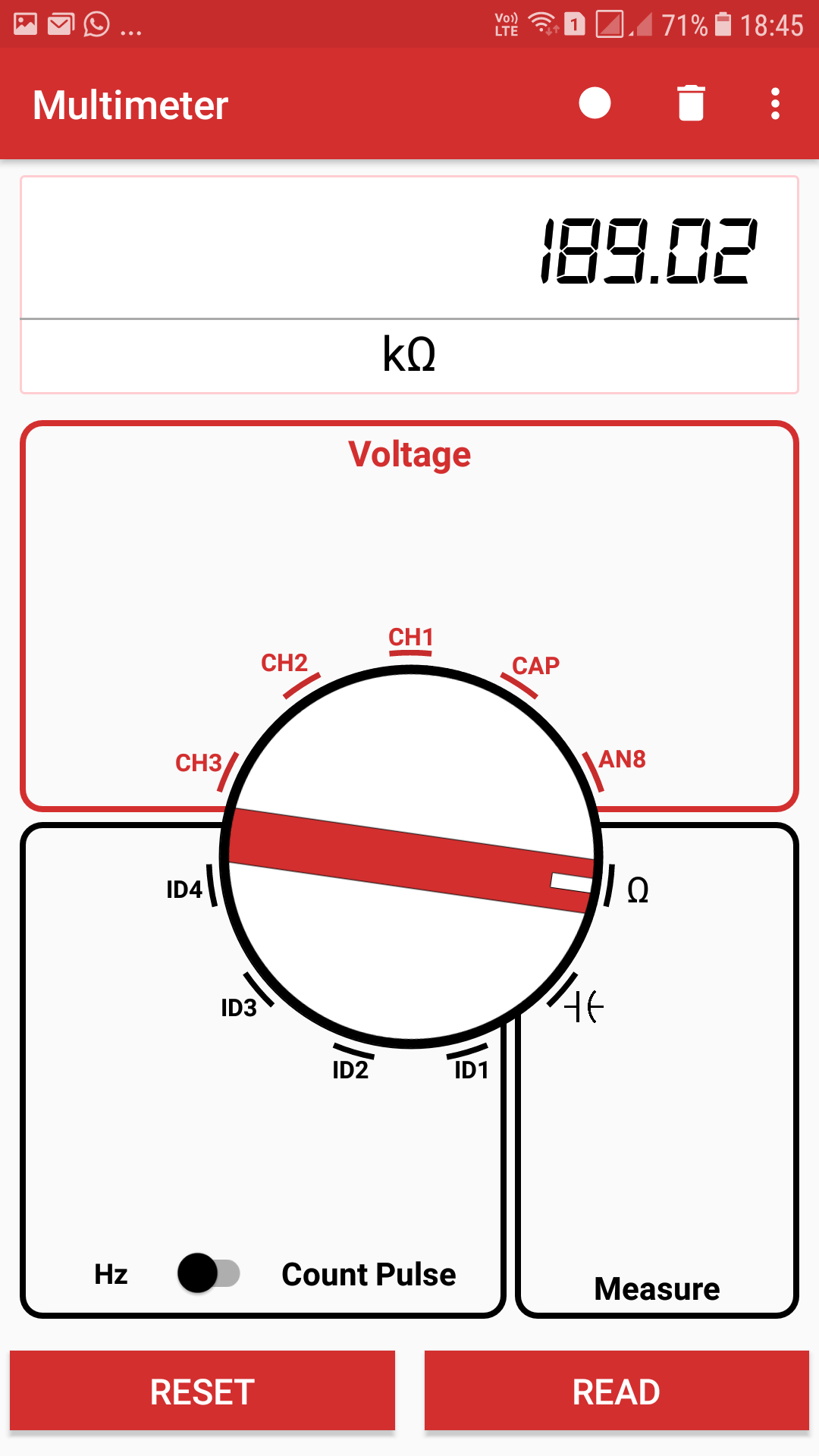Figure (1): Showing the implementation of multimeter toolbar

• Backend

For implementing the toolbar the onCreatemenu  was implemented for multimeter

```@Override
return true;
}```

For the same a separate menu was created for multimeter

```<?xml version="1.0" encoding="utf-8"?>
xmlns:app="http://schemas.android.com/apk/res-auto">
<item
android:id="@+id/record_pause_data"
android:icon="@drawable/record_icon"
android:title="@string/record_csv_data"
app:showAsAction="always" />
<item
android:id="@+id/delete_csv_data"
android:icon="@drawable/delete_icon"
android:title="@string/delete_csv_data"
app:showAsAction="ifRoom" />
<item
android:id="@+id/record_csv_data"
android:title="@string/save_csv_data"
app:showAsAction="never" />
<item
android:id="@+id/settings"
android:title="@string/nav_settings"
app:showAsAction="never" />Figure(2): Showing Toolbar of Multimeter

Thus through this the basic UI of the toolbar was implemented.

### Implementing the Data Export Feature

After implementing the toolbar for multimeter the multimeter the data exporting feature was implemented through the toolbar. The data export feature was implemented in such a manner that the user can start data recording by clicking on the record button after which the recording starts.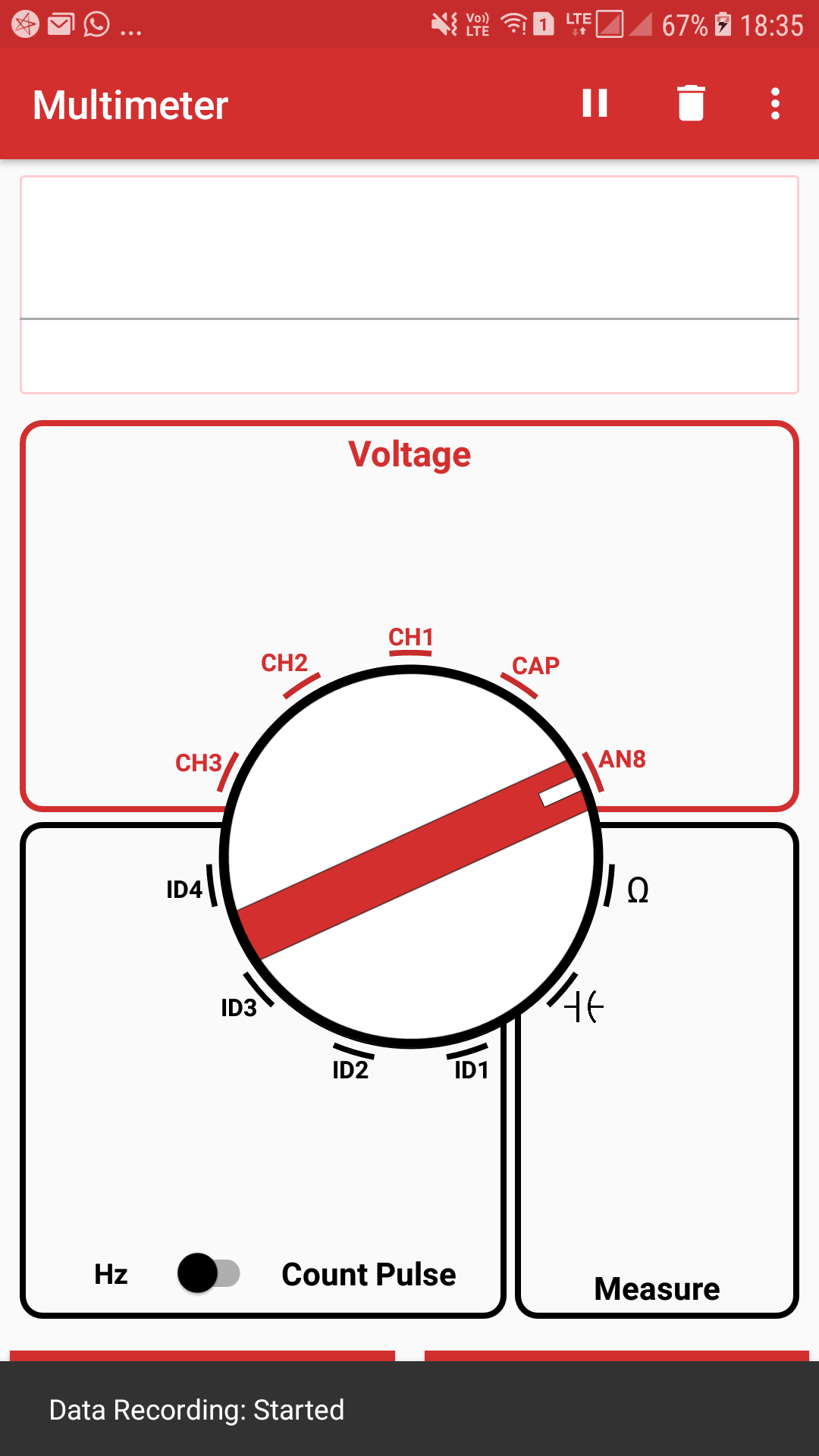Figure(3):Showing snackbar after the data recording is started

and then when the user wants to end the experiment he may click on the pause button after which he gets an option either to export the data in csv format or either to delete the data.Figure(4):Showing the menu bar for exporting data in csv

After clicking on the export data button the snacker appears which finally tells the user that the data is exported in this particular location (mentioned in the snackbar)Figure(5):Showing the snackbar for showing location of the CSV folder

Further going to the csv folder we get the excel sheet in which the data is exportedFigure(6):Showing the excel sheet of data recorded

And this is how the data exporting feature was implemented in the PSLab android app. The users can use this functionality to perform experiments using multimeter in PSLab.

### Resources

1. Medium, Android Toolbar for AppCompatActivity:
https://medium.com/@101/android-toolbar-for-appcompatactivity-671b1d10f354
2. Code Project, Exporting data in CSV format:

Continue Reading Implementing Toolbar and Exporting Data in CSV format in Multimeter

## Measuring Current in PSLab Android App

The Pocket Science Lab Android app has got various functionality such as voltmeter, resistance measurement, capacitance measurement, frequency measurement as well as Count pulse measurement , one of the missing functionality among these is the Ammeter, currently in PSLab there is no direct way of measuring current. In this blog I will be discussing an indirect method of measuring current in PSLab.

## Basics of measuring current

Generally in all multimeters , current is measured using  an Ammeter which uses the property of galvanometer to measure to measure current in PSLab. But as the PSLab doesn’t has any such embedded Galvanometer we cannot have a seperate Ammeter in it, but there is another method to measure the current, which is using the famous OHm’s law i.e V/I = R

In PSLab we can measure the voltage across any elements, plus we can also measure  the resistance of any circuit element in PSLab, the theory used for measuring current is stated as follows.

1. We connect the current-source to any known resistant(or any resistance) and then measure the voltage across the resistor.
2. Finally using ohm’s law the current will be voltage / resistance.

## Step-by-step guide on measuring the current

Here is the step by step guide on how to measure the current in PSLab:-

1. Take any arbitrary resistor and measure it’s resistance. To measure the resistance follow these steps :-
1. Take a resistance and connect it to any two pins of breadboard
2. Connect one end to the sen pin and the other end to the GND pin of PSLab device.
3. Now go to the Android app and select multimeter instrument.
4. Now in the multimeter instrument set the knob to resistance, in the measure section
5. Now click on the read button and then it will show the resistance of the resistor
2. Now that you have measured the resistance of the resistor, now connect the current source across the resistors.
3. Now finally measure the voltage across the resistor. To measure the voltage follow these steps.
1. Take two male to male wires and connect it’s two individual ends to the two ends of the resistor.
2. Now connect one of the other two ends of the wire to the any one of the CH1, CH2 or CH3 and the other end to GND respectively.
3. Now go to the Android app and select multimeter instrument.
4. Now in the multimeter instrument set the knob to the channel which you have selected i.e CH1, CH2 or CH3 , in the voltage section.
5. Now click on the read button and then it will show the voltage across the resistor.
4. Now using the using ohm’s law the current will be voltage / resistance.

Thus using these steps one can find the current in PSLab Android App.

## Images for the ExperimentFigure (1): Showing resistance measurementFigure(2) Showing voltage measurement

## Resources

1. Using-a-Multimeter, dengarden.com:
https://dengarden.com/home-improvement/Using-a-Multimeter
2. Wikipedia, Ohm’s Law:
https://en.wikipedia.org/wiki/Ohm%27s_law
Continue Reading Measuring Current in PSLab Android App

## Implementing Layouts for Different Screens in Android PSLab App

The Pocket Science Lab Android app has various functionalities implemented in it, one of the major implemented functionalities is the knob in the multimeter, In my previous blog I have mentioned about the knobs and how exactly it has been implemented, one the major problems in the knob is that it doesn’t fits correctly in all layouts, even though constraint layout is used , as it has different text-views positioned in a circular constraint in which exact circular radius has to be mentioned (which cannot be constrained), mentioned in my previous-blog there comes the idea of creating different layouts for different-screens in android.

### Need to create multiple layouts for different screen

There arises this question of the use of creating multiple layouts for different screens when you can create a flexible layout using tools such as constraint layout. The answer to this question is that in some cases such as as the case of circular constraint or using a linear-layout  one has to use use hard-coded dimensions which thus makes difference in various screens on, there comes the need of using size and orientation quantifiers i.e making layout according to screen sizes. AlsFigure 1:Screenshot

Figure 1 shows how different layouts are made for multimeter according to the screen-size.

### Size quantifiers in android

Size quantifier is a name that specifies an individual configuration (in this case size) corresponding to which resources can be used.

For example, here are some default and alternative resources:

```res/
drawable/
icon.png
background.png
drawable-hdpi/
icon.png
background.png```

In this case -hdpi is a size quantifier, there are default size quantifiers at the drawable folder.

### Making different layouts according to size-quantifiers

This blog will give a step by step guide on how to make layouts using size quantifiers.

1. Right click the res folder in android studio and select New-> Android Resource directory.
2. A screen like below will appear3. Change the resource type to layout.
4. From the available quantifiers select the quantifier which you would require, (in our case it is size).
5. After selecting the quantifier click the >> button after which the following screen will get displayed.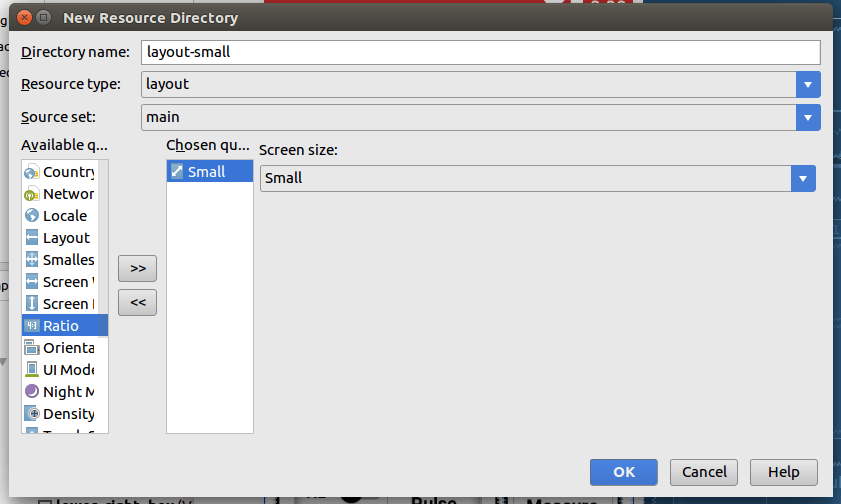6. Now select the screen-size and click ok to make the directory, note that only one directory can be made in a single go.
7. After this make different layouts according to the screen-sizes and then save the respective alternative resources in this new directory. The resource files must be named exactly the same as the default resource files.

Thus by following these steps one can make different layouts according to screen-sizes in android.

### Resources

2. App resources overview, Android developers https://developer.android.com/guide/topics/resources/providing-resources#ScreenSizeQualifier
Continue Reading Implementing Layouts for Different Screens in Android PSLab App

## Implementing Custom Rotary Knobs and Circular Positioning in the Multimeter in the PSLab Android App

In my previous blog [2I have discussed about how to implement  normal rotary knob using an open source library, this blog will be about the new user interface (UI) of multimeter in the PSLab Android app, how a custom rotary knob is implemented in it and how how the text views are positioned circular in them.

Implementation of Custom Rotary Knob

In the PSLab device  the rotary knob is implemented using the BeppiMenozzi Knob library as by doing this we don’t have to manually create the extra class for the knob and we don’t have to write the code from scratch.Figure 1: A basic rotary knob

Figure 1 shows a basic knob implemented using the BeppiMenozzi library whereas figure 2 shows the implementation of a custom knob using the basic knob.Figure 2: A custom Knob

Steps of making a Custom-Knob using a simple Knob

1. Implement the the basic knob using the steps given in my previous knobs explained in my previous blogs.
2. Download the images of the knob which has to be implemented.
```android:layout_weight="1"
android:rotation="15"
app:kDefaultState="2"
app:kIndicatorWidth="@dimen/multimeter_length_0"
app:kKnobCenterColor="@color/colorPrimaryDark"
app:kKnobColor="@color/white"
app:kKnobDrawable="@drawable/knob"```
1. Using the above code amend the knob as per the requirement. The advantage of using the beppiMonzi library is that the knob is fully amenable  , we can even define the minimum and maximum angle and many more stuffs can be done using the library.Figure 3: Showing the implementation of other custom knobs

The above figure shows the example of custom knobs implemented using the simple knob and by following the steps.

Implementation Circular positioning

One of the other major issues while making the new UI of the multimeter is the positioning of text-view around the circular knob. The issue was made overcome by implementing a circular positioning constraints in the text-views.

Steps of implementing circular positioning

1. Use the constraint layout version 1.1.0 or above as the previous versions do not support the circular positioning feature.
2. Add the circular constraint individually to every text-view.
```app:layout_constraintCircle="@id/knobs"
app:layout_constraintCircleAngle="105"

```

The above code snippets shows the addition od circular constraints added to a text-view. Using these constraint it decides positions the views relative to another views at a particular angle which thus makes up circular positioning.

Thus, this is how we can implement circular positioning in the views.

## Resources

1. BeppiMenozzi Knob Library
https://github.com/BeppiMenozzi/Knob
2. Rotary knob Blog
Continue Reading Implementing Custom Rotary Knobs and Circular Positioning in the Multimeter in the PSLab Android App

## Measuring capacitor in PSLab and its Bugs

In this blog I will discuss about how we have measured capacitance in Pocket Science Lab and the  issues in capacitance measurement which was finally solved.

Measuring capacitance in PSLab device

To measure capacitance we need to go to the multimeter instrument from the instrument section of the PSLab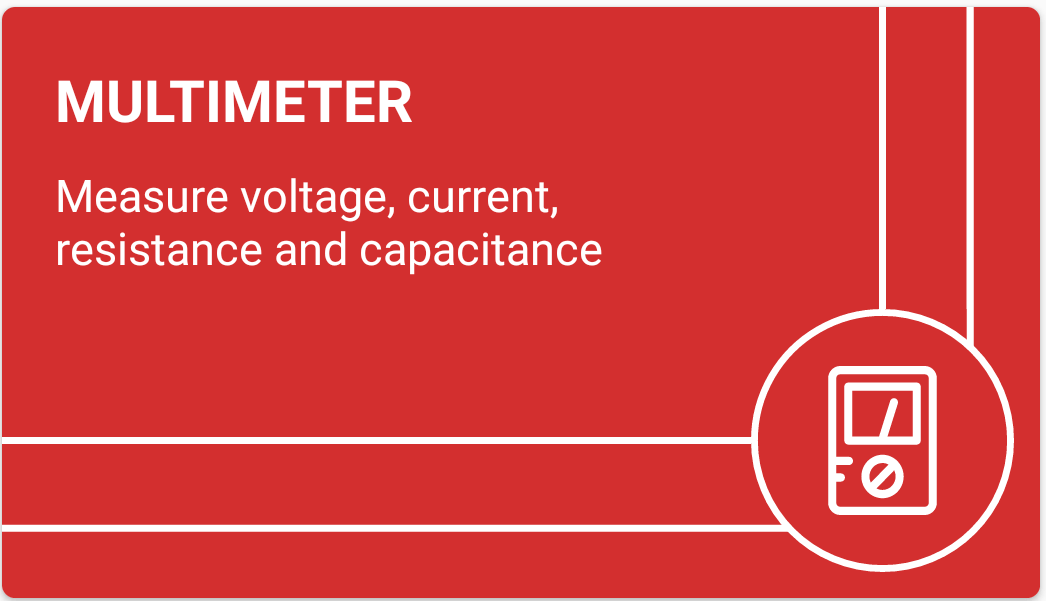Figure 1.  Showing Multimeter Tile

Capacitance in PSLab is measured by keeping the capacitor or the element of which capacitance is to be measured between the CAP and ground pin.Figure 2.  Showing CAP pins in PSLab

For measuring capacitance in PSLab we use a specific method in which we supply a constant current to the CAP pin and thus we charge the capacitor to the maximum level, the math involved in it is as follow:-

We know that

Q{charge stored} = C*V

Also

Q= I * time

Where

I=current (constant)

Thus the capacitance

C = Q / V

Therefore

C = I*time / V (measured). – (1)

Therefore we know the current supplied, we know the voltage measured and we have also set the time to charge the capacitor and thus we get the capacitance from equation (1).

Code implementation for measuring capacitance

This is the primary code for getting the data for measuring capacitance in which we pass the current range and the current time through which the data gets fetched from the device which is then further processed in another function in which we finally get the capacitance.

```public double[] getCapacitance(int currentRange, int chargeTime) { // time in uSec
try {
mPacketHandler.sendByte(mCommandsProto.COMMON);
mPacketHandler.sendByte(mCommandsProto.GET_CAPACITANCE);
mPacketHandler.sendByte(currentRange);
mPacketHandler.sendInt(chargeTime);
int VCode;
do VCode = mPacketHandler.getVoltageSummation();
while (VCode == -1);
double v = 3.3 * VCode / 4095;
mPacketHandler.getAcknowledgement();
double chargeCurrent = this.currents[currentRange];
double c = 0;
if (v != 0)
c = (chargeCurrent * chargeTime * 1e-6 / v - this.SOCKET_CAPACITANCE) / this.currentScalars[currentRange];
return new double[] {
v,
c
};
} catch (IOException | InterruptedException e) {
e.printStackTrace();
}
return null;```

In the above function we can clearly see how we send the bytes in the device by the sendByte function through which various functions are sending current, setting voltage, setting current range etc are done in the device and then we can see how the voltage measured is taken using the getVoltageSummition method (of packet Handler class) , how we get the current and finally using them in equation (1) we get the capacitance of the element.

The following implementation is taken from the PSLab desktop app where the same method is used to measure capacitance.

Bugs in measuring capacitance

The capacitance measurement although was working in the desktop app but had bugs in the android app. It could never read the correct value also everytime gave a null value for capacitance.Figure 3.  Showing null value for capacitance  PSLab

Solving the Bug 

After a deep research in the inside  the code of the capacitance measurement it was found that the error was caused while fetching the incorrect data from the device and processing it. The device gives a negative one value when there is any error in capacitance measurement  and that was being processed, thus the error was solved by introducing a do while loop as shown

``` do VCode = mPacketHandler.getVoltageSummation();
while (VCode == -1);```

And thus now only the correct data fetched is processed further and thus the bug was solved after which the capacitance measurement was correct.

## Resources

Continue Reading Measuring capacitor in PSLab and its Bugs

## Channel Communications Error of PSLab

The Pocket Science Lab multimeter has got three channels namely CH1,CH2 and CH3 with different ranges for measuring the voltages, but there was a channel communication error occuring at CH1 and CH2 pins of PSLab. This blogs will give a brief description of the channel communication error and how was it solved by me.

In the previous blog I have discussed about the channel communication of PSLab and how it works. In this blog i will be discussing about the channel communication error which was occuring while using CH1 and CH2 pins of PSLab android.

Communication between PSLab device and Android App

As discussed in the previous blog the communication between the PSLab and the android occurs with the help of the USBManger class of android.

One of the major function which makes it possible is the bulk transfer function of the android

`amtWritten = mConnection.bulkTransfer(mWriteEndpoint, src, written, writeLength, timeoutMillis);`

As shown in the above code, there is a timeout that some time required for this function to be executed, and otherwise this function will return a negative value which will mean that the communication is not successful.

Voltage Measuring Functions

The main function which gets called while pressing the button for measuring voltage is the getVoltage function which simultaneously calls the volmeterAutoRange function as well as the getAverage voltage function. The voltageAutoRnge function also calls the getAverage function inside of it.

```public double getVoltage(String channelName, Integer sample) {
this.voltmeterAutoRange(channelName);
double Voltage = this.getAverageVoltage(channelName, sample);
if (channelName.equals("CH3")) {
return Voltage;
} else {
return 2 * Voltage;
}
}```

Calling both these functions simultaneously results in calling of the bulktranfer method

VoltmeterAutoRange function:-

```private double voltmeterAutoRange(String channelName) {
return 0;
this.setGain(channelName, 0, true);
double V = this.getAverageVoltage(channelName, null);
return this.autoSelectRange(channelName, V);
}```

The getAverage voltage function calls the getRawableVoltage function which thus calls the USBManger class functions of read and write, thus calling the bulkTranfer function.

Thus as the bulk transfer function is called simultaneously it caused problem in communication.

Solving the issue

The communication related  issues were finally solved when these bugs were spotted, the solution to this issue is that the voltageAutoRange function’s return value was never used in the codes and was thus not required.The voltageAutoRange function was calling the getAverageVoltage function just to get a return value. Thus I formatted the function and now it looks like this-

```private void voltmeterAutoRange(String channelName) {
this.setGain(channelName, 0, true);
}
}```

And thus finally the issue was solved and all things were working fine in channel communication.

## Resources

Continue Reading Channel Communications Error of PSLab

## Voltage Measurement through Channels in PSLab

The Pocket Science Lab multimeter has got three channels namely CH1,CH2 and CH3 with different ranges for measuring the voltages.This blog will give a brief description on how we measure voltages in channels.

Measuring Voltages at channels can be divided into three parts:-

1. Communication between between device and Android.
2. Setting up analog channel (analog constants)
3. Voltage measuring function of android.

Communication between PSLab device and Android App

The communication between the PSLab device and  Android occurs through the help of UsbManger package of CommunicationHandler class of the app. The main two functions involved in the communication are read and write functions in which we send particular number of bytes and then we receive certain bytes.

```public int read(byte[] dest, int bytesToBeRead, int timeoutMillis) throws IOException {
} else {
}
}
//}
}```

Similarly the write function is –

```public int write(byte[] src, int timeoutMillis) throws IOException {
if (Build.VERSION.SDK_INT < 18) {
return writeSupportAPI(src, timeoutMillis);
}
int written = 0;
while (written < src.length) {
int writeLength, amtWritten;
//synchronized (mWriteBufferLock) {
writeLength = Math.min(mWriteBuffer.length, src.length - written);
// bulk transfer supports offset from API 18
amtWritten = mConnection.bulkTransfer(mWriteEndpoint, src, written, writeLength, timeoutMillis);
//}
if (amtWritten < 0) {
throw new IOException("Error writing " + writeLength +
" bytes at offset " + written + " length=" + src.length);
}
written += amtWritten;
}
return written;
}```

Although these are the core functions used for communication but the data received through these functions are further processed using another class known as PacketHandler. In the PacketHandler class also there are two major functions i.e sendByte and getByte(), these are the main functions which are further used in other classes for communication.

The sendByte function:-

```public void sendByte(int val) throws IOException {
if (!connected) {
throw new IOException("Device not connected");
}
try {
mCommunicationHandler.write(new byte[] {
(byte)(val & 0xff), (byte)((val >> 8) & 0xff)
}, timeout);
} catch (IOException e) {
Log.e("Error in sending int", e.toString());
e.printStackTrace();
}
} else {
burstBuffer.put(new byte[] {
(byte)(val & 0xff), (byte)((val >> 8) & 0xff)
});
}
}```

As we can see that in this function also the main function used is the write function of communicationHandler but in this class the data is further processed.

Setting Up the Analog Constants

For setting up the ranges, gains and other properties of channels, a different class of AnalogConstants is implemented in the android app, in this class all the properties which are used by the channels are defined which are further used in the sendByte() functions for communication.

```public class AnalogConstants {

public double[] gains = {1, 2, 4, 5, 8, 10, 16, 32, 1 / 11.};
public String[] allAnalogChannels = {"CH1", "CH2", "CH3", "MIC", "CAP", "SEN", "AN8"};
public String[] biPolars = {"CH1", "CH2", "CH3", "MIC"};
public Map<String, double[]> inputRanges = new HashMap<>();
public Map<String, Integer> picADCMultiplex = new HashMap<>();

public AnalogConstants() {

inputRanges.put("CH1", new double[]{16.5, -16.5});
inputRanges.put("CH2", new double[]{16.5, -16.5});
inputRanges.put("CH3", new double[]{-3.3, 3.3});
inputRanges.put("MIC", new double[]{-3.3, 3.3});
inputRanges.put("CAP", new double[]{0, 3.3});
inputRanges.put("SEN", new double[]{0, 3.3});
inputRanges.put("AN8", new double[]{0, 3.3});

}
}
```

Also in the AnalogInput sources class many other properties such as CHOSA( a variable assigned to denote the analog to decimal conversion constant of each channel) are also defined

```public AnalogInputSource(String channelName) {
AnalogConstants analogConstants = new AnalogConstants();
this.channelName = channelName;
range = analogConstants.inputRanges.get(channelName);
gainValues = analogConstants.gains;
calPoly10 = new PolynomialFunction(new double[] {
0.,
3.3 / 1023,
0.
});
calPoly12 = new PolynomialFunction(new double[] {
0.,
3.3 / 4095,
0.
});
if (range - range < 0) {
inverted = true;
inversion = -1;
}
if (channelName.equals("CH1")) {
gainEnabled = true;
gainPGA = 1;
gain = 0;
} else if (channelName.equals("CH2")) {
gainEnabled = true;
gainPGA = 2;
gain = 0;
}
gain = 0;
regenerateCalibration();
}```

Also in this constructor a polynomial function is also called which further plays an important  role in measuring voltage as it is through this polynomial function we get the voltage of channels in the science lab class , also it is also used in oscilloscope for plotting the graph . So this was the setup of analog channels.

Voltage Measuring Functions

There are two major functions for measuring voltages which are present in the scienceLab class

• getAverageVoltage
• getRawableVoltage

Here are the functions

```private double getRawAverageVoltage(String channelName) {
try {
int chosa = this.calcCHOSA(channelName);
mPacketHandler.sendByte(mCommandsProto.GET_VOLTAGE_SUMMED);
mPacketHandler.sendByte(chosa);
int vSum = mPacketHandler.getVoltageSummation();
mPacketHandler.getAcknowledgement();
return vSum / 16.0;
} catch (IOException | NullPointerException e) {
e.printStackTrace();
Log.e(TAG, "Error in getRawAverageVoltage");
}
return 0;
}```

This is the major function which takes the data from the communicationHandler class via packetHandler. Further this function is used in the getAverageVoltage function.

```private double getAverageVoltage(String channelName, Integer sample) {
if (sample == null) sample = 1;
PolynomialFunction poly;
double sum = 0;
ArrayList < Double > vals = new ArrayList < > ();
for (int i = 0; i < sample; i++) {
}
for (int j = 0; j < vals.size(); j++) {
sum = sum + poly.value(vals.get(j));
}
return sum / vals.size();
}```

This function uses the data from the getRawableVoltage function and uses it the polynomial generated in the analog lasses to calculate the final voltage. Thus this was the core backend of calculating the voltages through channels in PSLab.

Resources:

Continue Reading Voltage Measurement through Channels in PSLab

## Using Multimeter in PSLab Android Application

The Pocket Science Lab as we all know is on the verge of development and new features and UI are added almost every day. One such feature is the implementation of multimeter which I have also discussed in my previous blogs.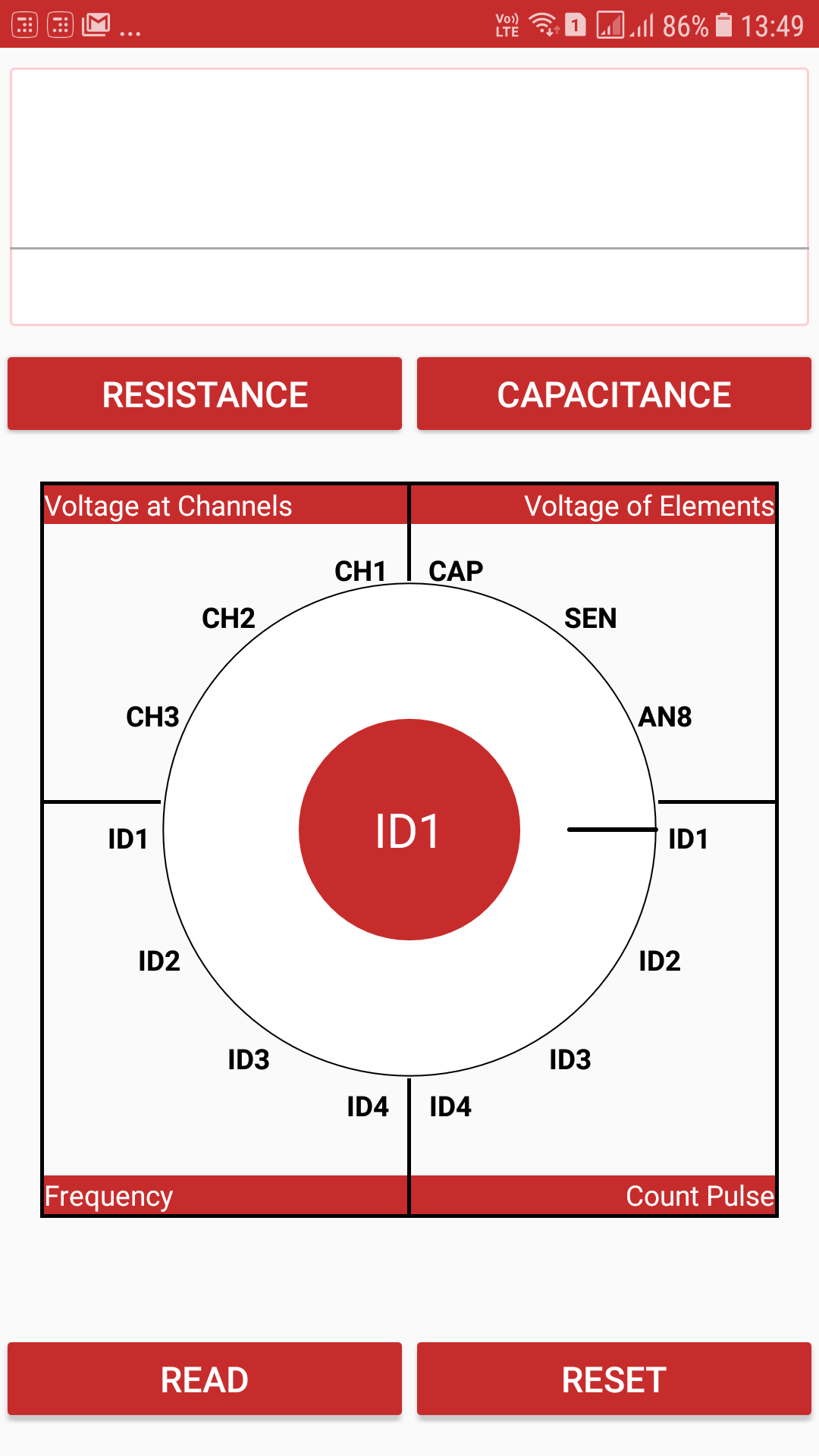Figure (1) : Screenshot of the multimeter

But although many functionality of multimeter such as resistance measurement are working perfectly, there are still various bugs in the multimeter. This blog is dedicated to using multimeter in the android app.

### Using the multimeter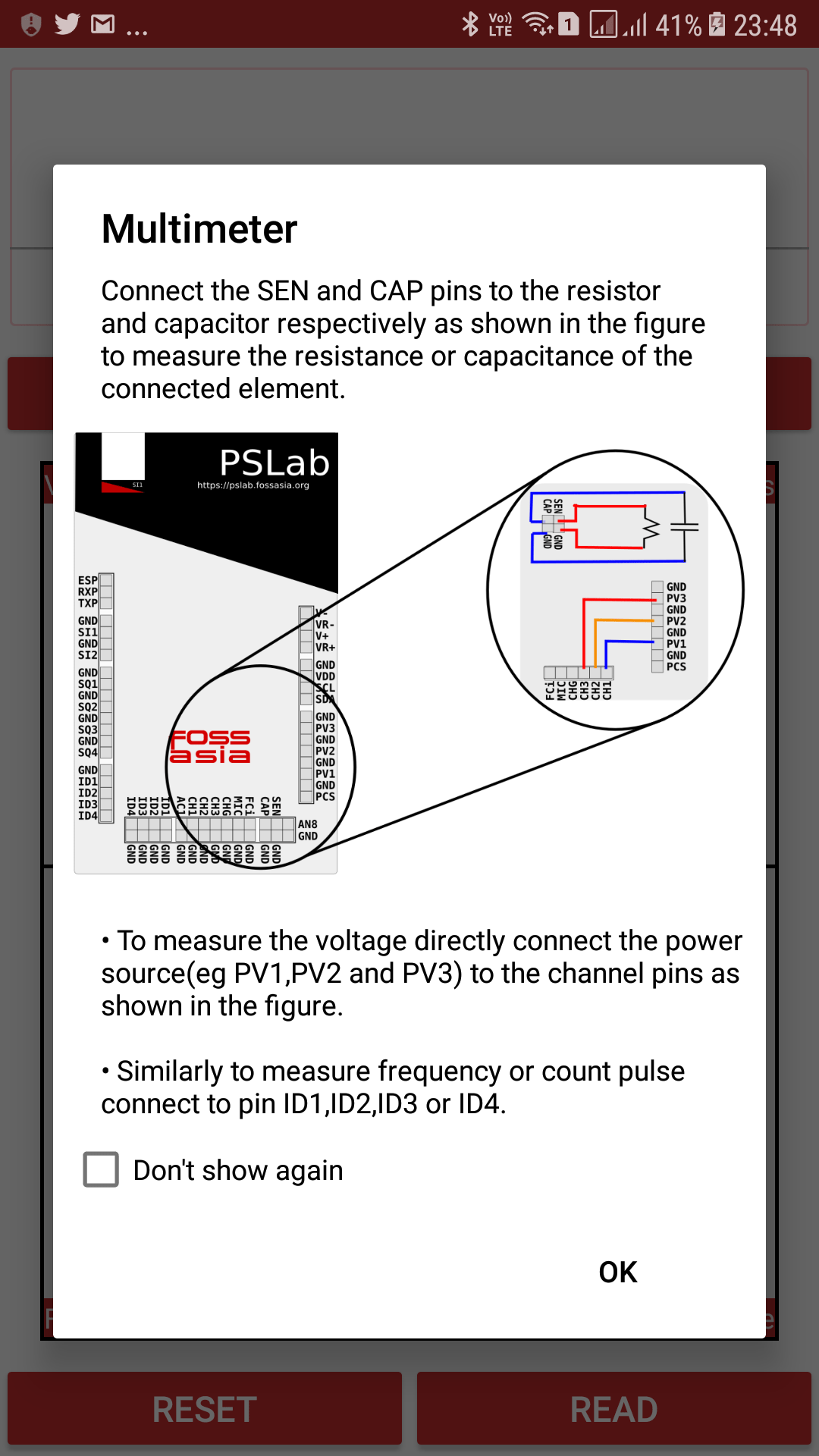Figure (2): Screenshot showing guide of multimeter

Figure (2) shows the guide of the multimeter, i.e how basic basic elements such as resistance and voltage are measured using the multimeter. The demonstration of measuring the resistance and voltage are given below.

#### Measuring the resistance

The resistance is measure by connecting the SEN pin to the positive end of resistor and the GND pin to the negative end of resistor and then clicking the RESISTANCE button.Figure (3) : Demonstration of resistance measurement

#### Measuring the voltage

To measure the voltage as said in the Guide, directly connect the power source to the the channel pins, although currently only the CH3 pin will show the most accurate results, work is going on other channel improvisation as well.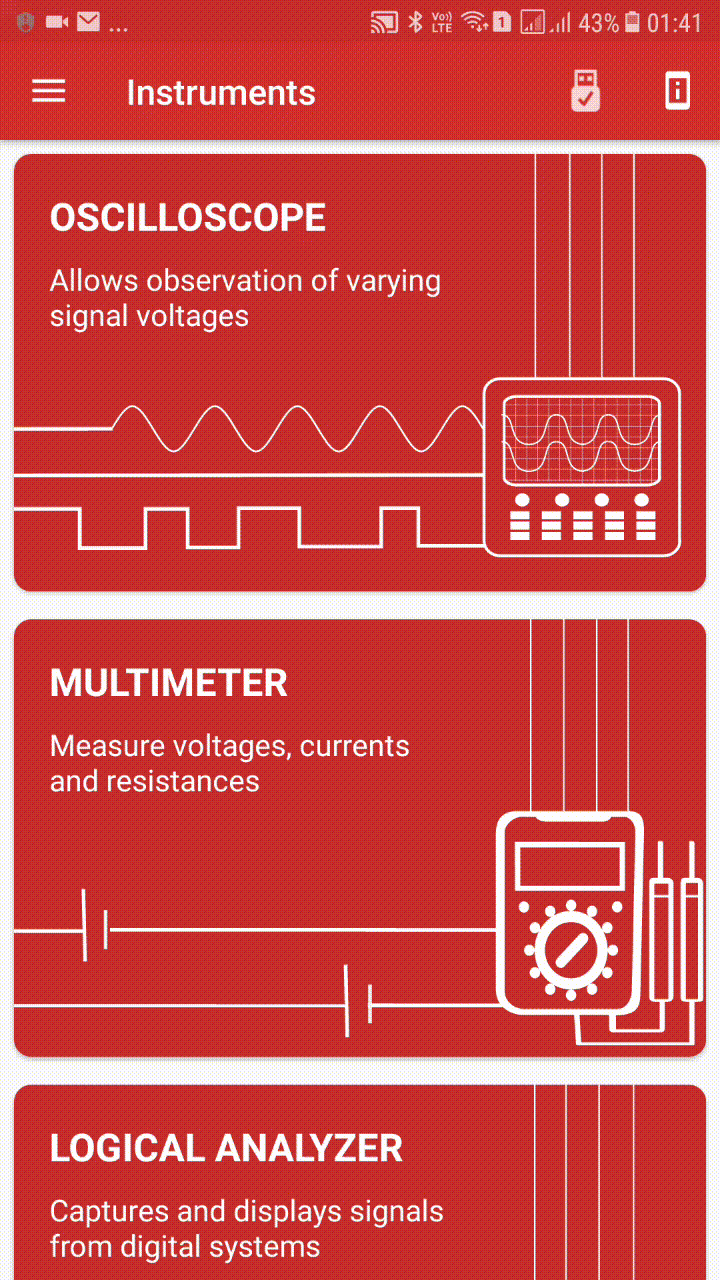Figure (4) : Demonstration of Voltage measurement

And thus this is how the multimeter is used is used in the android app.  Of course there are still many many features such as capacitance measurements which are yet to be implemented and the work is going on them

Resources:

Continue Reading Using Multimeter in PSLab Android Application

## Implementing Multimeter in PSLab Android App

The Pocket Science Lab Android app being on the verge of development have various new features adding up per day. One of the new things added up recently is the splitting of the control section in three different instruments and implementing the control read section into a multimeter. This blog will be discussing about how the multimeter is implemented.

The different instruments are power section, multimeter and wave generator. While in the previous implementation of control section it was divided into three parts namely control main, control read and control advanced as shown in figure (1). The control is the power source, read is the multimeter and advanced section is the wave generator.Figure  (1): Screenshot of control section

Figure (1) shows the previous implementation of a multimeter i.e the read section but as we know this is way different than the actual implementation of a multimeter and thus from here comes the task of implementing a new multimeter.

What is a Multimeter, how does it looks?

A multimeter basically is an instrument designed to measure electric current, voltage, and usually resistance, typically over several ranges of value.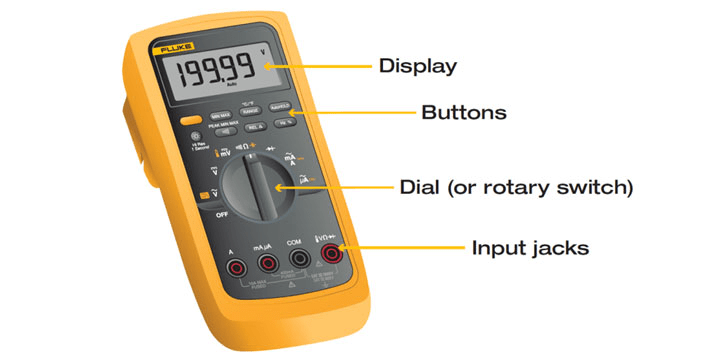Figure (2): Showing a real multimeter instrument and its different sections 

Figure(2) clearly shows how an actual multimeter looks. It basically has three important components i.e the display the buttons and the rotary knob or the dial and thus the task was to implement the same in PSLab android.

Implementation in PSLabFigure (3) :  Screenshot of new implementation of multimeter

The implementation of multimeter is thus inspired from its original look i.e it has got basic buttons, a rotary knob and a display. Figure (3) shows the implementation of multimeter in the android-app

Back-end of Multimeter

A separate multimeter activity was implemented for the multimeter. The main back-end part of getting the resistance, capacitance, frequency and count pulse were taken from the communication related classes such as the ScienceLab class and PacketHandler class. For example to get the voltage calculation we use the getRawableVoltage function

```private double getRawAverageVoltage(String channelName) {
try {
int chosa = this.calcCHOSA(channelName);
mPacketHandler.sendByte(mCommandsProto.GET_VOLTAGE_SUMMED);
mPacketHandler.sendByte(chosa);
int vSum = mPacketHandler.getVoltageSummation();
mPacketHandler.getAcknowledgement();
return vSum / 16.0;
} catch (IOException | NullPointerException e) {
e.printStackTrace();
Log.e(TAG, "Error in getRawAverageVoltage");
}
return 0;
}```

The above function shows the pure backend of PSLab and how data is taken from the hardware using the packet handler class, after which the data is processed in various other functions after we getting the final result. Similarly the function to get count pulse is

```public int readPulseCount() {
try {
mPacketHandler.sendByte(mCommandsProto.COMMON);
mPacketHandler.sendByte(mCommandsProto.FETCH_COUNT);
int count = mPacketHandler.getVoltageSummation();
mPacketHandler.getAcknowledgement();
return 10 * count;
} catch (IOException e) {
e.printStackTrace();
}
return -1;
}```

As we see that the data is being taken through a similar manner in the above function i.e using the packetHandler class(by sending and receiving bytes). Thus in all the other functions for capacitance, frequency similar communication model can be found.

Similarly all the functions are implemented in the ScienceLab class and thus all the functions are directly called from the ScienceLab class in the Multimeter activity. For more knowledge on these one can directly have a look at the PSLab android app codes available in Github.

Implementation of the Rotary Knob

The rotary knob is implemented using the BeppiMenozzi Knob library. More information regarding the same can be found in my previous blog on implementing the rotary knob.

Resources:

Continue Reading Implementing Multimeter in PSLab Android App